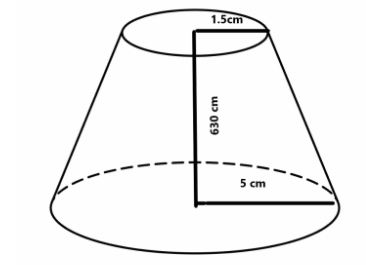Courses
Courses for Kids
Free study material
Offline Centres
MoreLast updated date: 04th Dec 2023
Total views: 280.2k
Views today: 5.80k

# The base and top radius of a truncated cone is 5cm and 1.5cm. And its height is 630 cm. What is the volume of a truncated cone?Verified
280.2k+ views
Hint: The volume of a cone is given by
$V = \dfrac{1}{3}(\pi {r^2}h) \;$ .
In our case it is given that the cone is truncated and the values of top and bottom radius are given.
To calculate the volume of a truncated cone we can use the formula.
$V{\text{ }} = \;\dfrac{{\pi h}}{3}({R^2} + Rr + {r^2})$

$V{\text{ }} = \;\dfrac{{\pi h}}{3}({R^2} + Rr + {r^2})$
$V{\text{ }} = \;\dfrac{{\pi (630)}}{3} \times [{(5)^2} + (5)(1.5) + {(1.5)^2}] \\ \Rightarrow 210\pi \times (25 + 7.5 + 2.25) \\ \Rightarrow 210\pi \times (34.75) \;$
By further solving we get Volume of the truncated cone = $22935\,c{m^3}$
So, the correct answer is “ $22935\,c{m^3}$ ”.